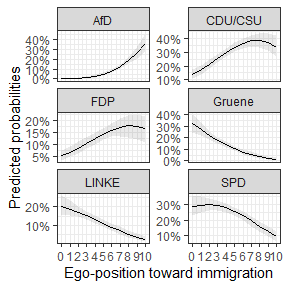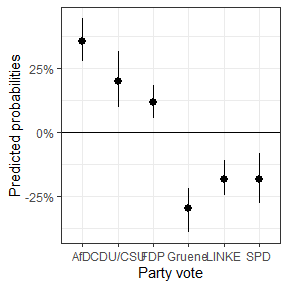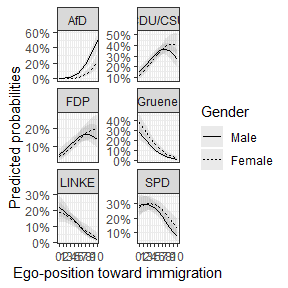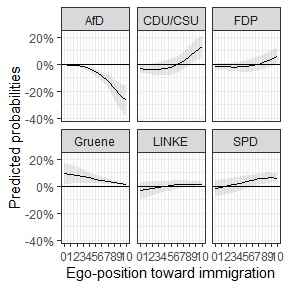# Observed Value Predictions for Multinomial Logit Models

This package provides functions that make it easy to get plottable predictions from multinomial logit models. The predictions are based on simulated draws of regression estimates from their respective sampling distribution.

At first I will present the theoretical and statistical background, before using sample data to demonstrate the functions of the package.

## The multinomial logit model

This is a short introduction in the theoretical and statistical background of the multinomial logit.

Dependent variables can not necessarily be ordered. In political science, for example, the variable of interest is often the individual’s vote choice, based on the set of parties that are presented. Of interest is then how somebody comes up with their choice.

More generally spoken, many questions deal with a nominal outcome variable and we want to test assumptions about the function that may lead to a respective outcome.

For these questions, the multinomial logit model is often a fitting option. Similar to an ordinary logit model, the multinomial logit model assumes that the probability to choose one over the other outcomes can be modeled with a linear function and a fitting logit link function. The difference of the multinomial logit is that it models the choice of each category as a function of the characteristics of the observation.

In formal terms, we assume $\Pr(y_i = j|X_i)$ is a linear combination of $X_i\beta_j$, whereby $\beta_j$ is a choice specific vector. This means we are interested in the probability that the observed choice of the individual $y_i$ is the choice category $j$ dependent on characteristics of the observation’s characteristics $X_i$. Therefore we estimate a choice specific vector $$\beta_j$$. Since the probability is restricted to be between $0$ and $1$, we use $exp(X_i\beta_j)$ as a fitting link function. Additionally, we bring the exponents into relationship with each other and normalize them by dividing through the sum of them.

Since we cannot compare all choices against each other, the model is not identified so far. Instead, we have to choose a baseline category and fix it to $0$. Therefore we estimate the probability of all choices to be chosen in comparison to the baseline choice.

Eventually, we end up with the following probability function:

$\Pr(y_i|X_i)= \frac{exp(X_i\beta_j)}{\sum^{J}_{m=1}exp(X_i \beta_m)}$, whereby $\beta_1 = 0$ This is the link function that is used for estimation.

For a more detailed insight into the multinomial model refer to sources like these lecture notes by Germán Rodríguez.

## Using the package

### How does the function work?

As we have seen above, the multinomial logit can be used to get an insight into the probabilities to choose one option out of a set of alternatives. We have also seen that we need a baseline category to identify the model. This is mathematically necessary, but does not come in handy for purposes of interpretation.

It is far more helpful and easier to understand to come up with predicted probabilities and first differences for values of interest (see e.g., King, Tomz, and Wittenberg 2000 for approaches in social sciences). Based on simulations, this package helps to easily predict probabilities and confidence intervals for each choice category over a specified scenario. The functions use the observed values to compute the predicted probabilities, as is recommended by Hanmer and Ozan Kalkan (2013).

The procedure follows the following steps:

1. Estimate a multinomial model and save the coefficients and the variance covariance matrix (based on the Hessian-matrix of the model).
2. To simulate uncertainty, make $$n$$ draws of coefficients from a simulated sampling distribution based on the coefficients and the variance covariance matrix.
3. Predict probabilities by multiplying the drawn coefficients with a specified scenario (so far these are the observed values).
4. Take the mean and the quantiles of the simulated predicted probabilities.

The presented functions follow these steps. Additionally, they use the so called observed value approach. This means that the “scenario” uses all observed values that informed the model. Therefore the function takes these more detailed steps:

1. For all (complete) cases $$n$$ predictions are computed based on their observed independent values and the $$n$$ sets of coefficients.
2. Next the predicted values of all observations for each simulation are averaged.
3. Take the mean and the quantiles of the simulated predicted probabilities (same as above).

For first differences, the simulated predictions are subtracted from each other.

To showcase these steps, I present a reproducible example of how the functions can be used.

### Example

The example uses data from the German Longitudinal Election Study (GLES, Roßteutscher et al. (2019)).

The contains 1,000 respondents characteristics and their vote choice.

For this task, we need the following packages:

# Required packages
library(magrittr) # for pipes
library(nnet) # for the multinom()-function
library(MASS) # for the multivariate normal distribution

# The package
library(MNLpred)

# Plotting the predicted probabilities:
library(ggplot2)
library(scales)

# The data:
data("gles")

The next step is to compute the actual model. The function of the MNLpred package is based on models that were estimated with the multinom()-function of the nnet package. The multinom() function is convenient because it does not need transformed datasets. The syntax is very easy and resembles the ordinary regression functions. Important is that the Hessian matrix is returned with Hess = TRUE. The matrix is needed to simulate the sampling distribution.

As we have seen above, we need a baseline or reference category for the model to work. Therefore, be aware what your baseline category is. If you use a dependent variable that is of type character, the categories will be ordered in alphabetical order. If you have a factorat hand, you can define your baseline category, for example with the relevel()function.

Now, let’s estimate the model:

# Multinomial logit model:
mod1 <- multinom(vote ~ egoposition_immigration +
political_interest +
income + gender + ostwest,
data = gles,
Hess = TRUE)
#> # weights:  42 (30 variable)
#> initial  value 1791.759469
#> iter  10 value 1644.501289
#> iter  20 value 1553.803188
#> iter  30 value 1538.792079
#> final  value 1537.906674
#> converged

The results show the coefficients and standard errors. As we can see, there are five sets of coefficients. They describe the relationship between the reference category (AfD) and the vote choices for the parties CDU/CSU, FDP, Gruene, LINKE, and SPD.

summary(mod1)
#> Call:
#> multinom(formula = vote ~ egoposition_immigration + political_interest +
#>     income + gender + ostwest, data = gles, Hess = TRUE)
#>
#> Coefficients:
#>         (Intercept) egoposition_immigration political_interest      income
#> CDU/CSU    3.101201              -0.4419104        -0.29177070  0.33114348
#> FDP        2.070618              -0.4106626        -0.19044703  0.18496691
#> Gruene     3.232074              -0.8482213        -0.03023454  0.24330589
#> LINKE      4.990008              -0.7477359        -0.04503371 -0.24206850
#> SPD        3.799394              -0.6425427        -0.03514426  0.08211066
#>           gender     ostwest
#> CDU/CSU 1.296949  0.79760035
#> FDP     1.252112  1.01378955
#> Gruene  1.831714  0.76299897
#> SPD     1.497019  0.74026388
#>
#> Std. Errors:
#>         (Intercept) egoposition_immigration political_interest    income
#> CDU/CSU   0.8568928              0.06504100          0.1694270 0.1872533
#> FDP       0.9508589              0.07083385          0.1882974 0.2063590
#> Gruene    0.9854950              0.07887427          0.1969704 0.2149368
#> LINKE     0.9505656              0.07755359          0.1962954 0.2058036
#> SPD       0.8880256              0.06912678          0.1779570 0.1924002
#>            gender   ostwest
#> CDU/CSU 0.3530225 0.3120178
#> FDP     0.3794295 0.3615071
#> Gruene  0.3875116 0.3671397
#> SPD     0.3625652 0.3269007
#>
#> Residual Deviance: 3075.813
#> AIC: 3135.813

A first rough review of the coefficients shows that a more restrictive ego-position toward immigration leads to a lower probability of the voters to choose any other party than the AfD. It is hard to evaluate whether the effect is statistically significant and how the probabilities for each choice look like. For this it is helpful to predict the probabilities for certain scenarios and plot the means and confidence intervals for visual analysis.

Let’s say we are interested in the relationship between the ego-position toward immigration and the probability to choose any of the parties. It would be helpful to plot the predicted probabilities for the span of the positions.

summary(gles$egoposition_immigration) #> Min. 1st Qu. Median Mean 3rd Qu. Max. #> 0.000 3.000 4.000 4.361 6.000 10.000 As we can see, the ego positions were recorded on a scale from 0 to 10. Higher numbers represent more restrictive positions. We pick this score as the x-variable (x) and use the mnl_pred_ova() function to get predicted probabilities for each position in this range. The function needs a multinomial logit model (model), data (data), the variable of interest x, the steps for which the probabilities should be predicted (by). Additionally, a seed can be defined for replication purposes, the numbers of simulations can be defined (nsim), and the confidence intervals (probs). If we want to hold another variable stable, we can specify so with zand z_value. See also the mnl_fd_ova() function below. pred1 <- mnl_pred_ova(model = mod1, data = gles, x = "egoposition_immigration", by = 1, seed = "random", # default nsim = 100, # faster probs = c(0.025, 0.975)) # default #> Multiplying values with simulated estimates: #> ================================================================================ #> Applying link function: #> ================================================================================ #> Done! The function returns a list with several elements. Most importantly, it returns a plotdata data set: pred1$plotdata %>% head()
#>   egoposition_immigration vote        mean        lower       upper
#> 1                       0  AfD 0.002362157 0.0008889346 0.005310986
#> 2                       1  AfD 0.004536089 0.0019850282 0.009236804
#> 3                       2  AfD 0.008520298 0.0043845143 0.015691889
#> 4                       3  AfD 0.015608263 0.0091138279 0.025959243
#> 5                       4  AfD 0.027788172 0.0183414635 0.040928589
#> 6                       5  AfD 0.047864455 0.0350754281 0.064213068

As we can see, it includes the range of the x variable, a mean, a lower, and an upper bound of the confidence interval. Concerning the choice category, the data is in a long format. This makes it easy to plot it with the ggplot syntax. The choice category can now easily be used to differentiate the lines in the plot by using linetype = vote in the aes(). Another option is to use facet_wrap() or facet_grid() to differentiate the predictions:

ggplot(data = pred1$plotdata, aes(x = egoposition_immigration, y = mean, ymin = lower, ymax = upper)) + geom_ribbon(alpha = 0.1) + # Confidence intervals geom_line() + # Mean facet_wrap(.~ vote, scales = "free_y", ncol = 2) + scale_y_continuous(labels = percent_format(accuracy = 1)) + # % labels scale_x_continuous(breaks = c(0:10)) + theme_bw() + labs(y = "Predicted probabilities", x = "Ego-position toward immigration") # Always label your axes ;)If we want first differences between two scenarios, we can use the function mnl_fd2_ova(). The function takes similar arguments as the function above, but now the values for the scenarios of interest have to be supplied. Imagine we want to know what difference it makes to position oneself on the most tolerant or most restrictive end of the egoposition_immigration scale. This can be done as follows: fdif1 <- mnl_fd2_ova(model = mod1, data = gles, x = "egoposition_immigration", value1 = min(gles$egoposition_immigration),
value2 = max(gles$egoposition_immigration), nsim = 100) #> Multiplying values with simulated estimates: #> ================================================================================ #> Applying link function: #> ================================================================================ #> Done! The first differences can then be depicted in a graph. ggplot(fdif1$plotdata_fd, aes(x = categories,
y = mean,
ymin = lower, ymax = upper)) +
geom_pointrange() +
geom_hline(yintercept = 0) +
scale_y_continuous(labels = percent_format()) +
theme_bw() +
labs(y = "Predicted probabilities",
x = "Party vote")We are often not only interested in the static difference, but the difference across a span of values, given a difference in a second variable. This is especially helpful when we look at dummy variables. For example, we could be interested in the effect of gender on the vote decision over the different ego-positions. With the mnl_fd_ova() function, we can predict the probabilities for two scenarios and subtract them. The function returns the differences and the confidence intervals of the differences. The different scenarios can be held stable with z and the z_values. z_values takes a vector of two numeric values. These values are held stable for the variable that is named in z.

fdif2 <- mnl_fd_ova(model = mod1,
data = gles,
x = "egoposition_immigration",
by = 1,
z = "gender",
z_values = c(0,1),
nsim = 100)
#> First scenario:
#> Multiplying values with simulated estimates:
#> ================================================================================
#> ================================================================================
#> Done!
#>
#> Second scenario:
#> Multiplying values with simulated estimates:
#> ================================================================================
#> ================================================================================
#> Done!

As before, the function returns a list including a data set that can be used to plot the differences.

fdif2$plotdata_fd %>% head() #> egoposition_immigration vote mean lower upper #> 1 0 AfD -0.002663232 -0.005118217 -0.001010217 #> 2 1 AfD -0.005103397 -0.009651883 -0.002038105 #> 3 2 AfD -0.009549265 -0.017744719 -0.004345490 #> 4 3 AfD -0.017380503 -0.029006213 -0.008910537 #> 5 4 AfD -0.030614814 -0.049294753 -0.016816706 #> 6 5 AfD -0.051820127 -0.080766011 -0.030883162 Since the function calls the mnl_pred_ova() function internally, it also returns the output of the two predictions in the list element Prediction1 and Prediction2. The plot data for the predictions is already bound together row wise to easily plot the predicted probabilities. ggplot(data = fdif2$plotdata, aes(x = egoposition_immigration,
y = mean,
ymin = lower, ymax = upper,
group = as.factor(gender),
linetype = as.factor(gender))) +
geom_ribbon(alpha = 0.1) +
geom_line() +
facet_wrap(. ~ vote, scales = "free_y", ncol = 2) +
scale_y_continuous(labels = percent_format(accuracy = 1)) + # % labels
scale_x_continuous(breaks = c(0:10)) +
scale_linetype_discrete(name = "Gender",
breaks = c(0, 1),
labels = c("Male", "Female")) +
theme_bw() +
labs(y = "Predicted probabilities",
x = "Ego-position toward immigration") # Always label your axes ;)As we can see, the differences between female and male differ, depending on the party and ego-position. So let’s take a look at the differences:

ggplot(data = fdif2\$plotdata_fd, aes(x = egoposition_immigration,
y = mean,
ymin = lower, ymax = upper)) +
geom_ribbon(alpha = 0.1) +
geom_line() +
geom_hline(yintercept = 0) +
facet_wrap(. ~ vote, ncol = 3) +
scale_y_continuous(labels = percent_format(accuracy = 1)) + # % labels
scale_x_continuous(breaks = c(0:10)) +
theme_bw() +
labs(y = "Predicted probabilities",
x = "Ego-position toward immigration") # Always label your axes ;)We can see that the differences are for some parties at no point statistically significant from 0.

## Conclusion

Multinomial logit models are important to model nominal choices. They are, however, restricted by being in need of a baseline category. Additionally, the log-character of the estimates makes it difficult to interpret them in meaningful ways. Predicting probabilities for all choices for scenarios, based on the observed data provides much more insight. The functions of this package provide easy to use functions that return data that can be used to plot predicted probabilities. The function uses a model from the multinom() function and uses the observed value approach and a supplied scenario to predict values over the range of fitting values. The functions simulate sampling distributions and therefore provide meaningful confidence intervals. mnl_pred_ova() can be used to predict probabilities for a certain scenario. mnl_fd_ova() can be used to predict probabilities for two scenarios and their first differences.

## Acknowledgment

My code is inspired by the method courses in the Political Science master’s program at the University of Mannheim(cool place, check it out!). The skeleton of the code is based on a tutorial taught by Marcel Neunhoeffer (lecture: “Advanced Quantitative Methods” by Thomas Gschwend).